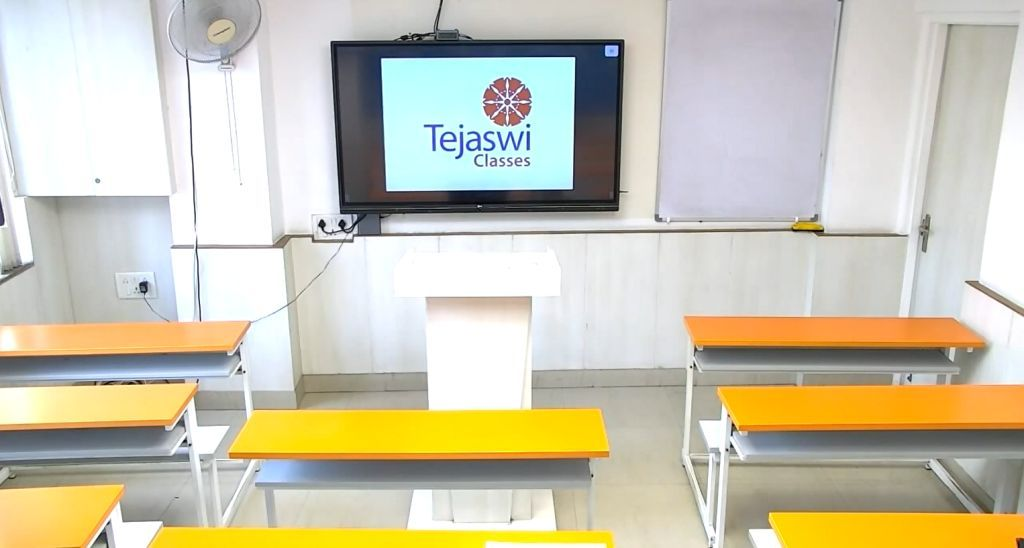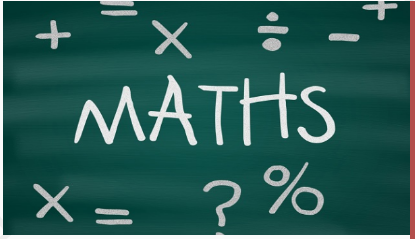# Tejaswi Classes is the Best coaching Institute for Maths Class X - XII & IIT JEE in Dwarka. Learn from Experts for IIT - JEE Mains and Advanced.

### Class XI - XII Maths Tuition Classes in Dwarka

Tejaswi Classes offers support for Mathematics for classes 12th.
Program Objectives

To acquire knowledge & critical understanding of the basic concepts, terms, principles, symbols & mastery of underlying processes & skills To feel the flow of reasons while proving a result or solving a problem To develop skills to think, analyze and articulate logically To acquaint students with different aspects of mathematics used I daily life### CALCULAS

Calculus is a branch of mathematics that involves the study of rates of change. Calculus helped to determine how particles, stars, and matter actually move and change in real time. Calculus is used in a multitude of fields that you wouldn’t ordinarily think would make use of its concepts.

### THREE DIMENSIONAL GEOMETRY

3 Dimensional geometry involves the mathematics of shapes in 3D space and involves 3 coordinates in the XYZ plane which are x-coordinate, y-coordinate and z-coordinate. The shapes that occupy space are called 3D shapes.

### ALGEBRA

Algebra is one of the broad areas of mathematics, together with number theory, geometry and analysis. In its most general form, algebra is the study of mathematical symbols and the rules for manipulating these symbols; it is a unifying thread of almost all of mathematics.

### TRIGONOMETRY

Trigonometry basics deal with the measurement of angles and problems related to angles. There are three basic functions in trigonometry: sine, cosine, and tangent. These three basic ratios or functions can be used to derive other important trigonometric functions: cotangent, secant, and cosecant.

### PROBABILITY

NCERT Solutions for Class 12 Maths All Chapters Probability is designed and prepared by the best teachers across India. All the important topics are covered in the exercises and each answer comes with a detailed explanation to help students understand concepts better.

### VECTORS

NCERT solutions for class 12 Maths Chapter 10 Vector Algebra exercise 10.1, 10.2, 10.3, 10.4 and miscellaneous exercises in Hindi and English Medium free PDF file format for UP Board and CBSE Board students to free download for 2021-22.

## Class 11th and 12th Maths Tutorials in Dwarka

What are the basic maths topics?
The major branches of maths are:

• Number system and basic Arithmetic.
• Algebra.
• Trigonometry.
• Geometry and Cartesian
• Geometry.
• Calculus- Differential and Integral.
• Matrix Algebra.
• Probability and Statistics.

What are the 5 different branches of mathematics?

• Image result for maths classes overview
• Here are the main branches of mathematics:
• Foundations.
• Arithmetic.
• Algebra.
• Geometry.
• Trigonometry.
• Calculus.
• Probability and Statistics.
• Number Theory.

### What is Maths standard?

The Standard exam is meant for students who wish to study Mathematics in higher classes, while the Mathematics Basic is taken by students who do not wish to pursue advanced Mathematics in higher classes. As a result, the level of difficulty of the Standard exam was way higher than the Basic exam.